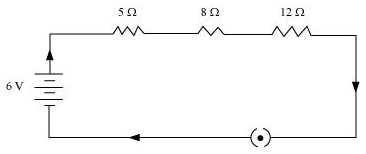Draw a schematic diagram of a circuit consisting of a battery of three cells of 2 V each, a 5 Ω resistor

Draw a schematic diagram of a circuit consisting of a battery of three cells of 2 V each, a 5 Ω resistor, an 8 Ω resistor, and a 12 Ω resistor, and a plug key, all connected in series.

verified

Three cells of potential 2 V, each connected in series therefore the potential difference of the battery will be 2 V + 2 V + 2 = 6V. The following circuit diagram shows three resistors of resistances 5 Ω, 8 Ω and 12 Ω respectively connected in series and a battery of potential 6 V and a plug key which is closed means the current is flowing in the circuit.